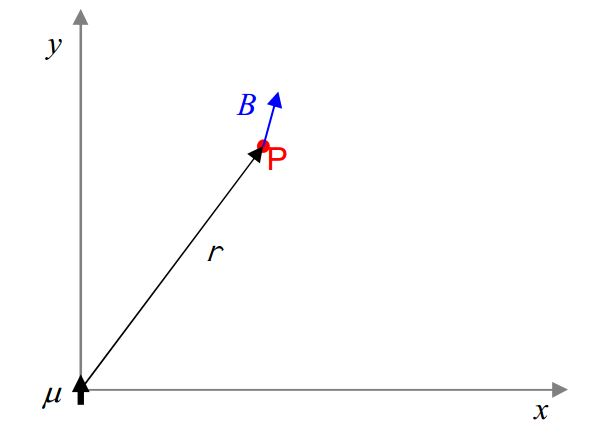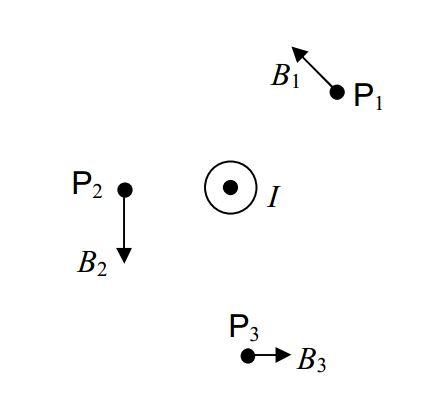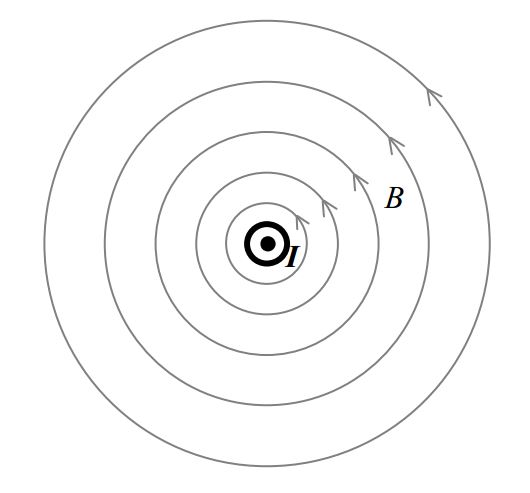$$\require{cancel}$$

# B17: Magnetic Field: Causes

This chapter is about magnetism but let’s think back to our introduction to charge for a moment. We talked about the electric field before saying much about what caused it. We said the electric field exerts a force on a particle that has charge. Later we found out that charged particles play not only the role of “victim” to the electric field but, that charged particles cause electric fields to exist.

Now we have been talking about the magnetic field. We have said that the magnetic field exerts a torque on a particle that has magnetic dipole moment. You might guess that a particle that has magnetic dipole moment would cause a magnetic field. You’d be right! A particle that has the physical property known as magnetic dipole moment causes a magnetic field to exist in the region of space around it. A magnetic field can be caused to exist by a particle having magnetic dipole moment or a distribution of particles having magnetic dipole moment.

The magnetic field at point $$P$$, an empty point in space in the vicinity of a particle that has a magnetic dipole moment, due to that particle-with-magnetic-dipole-moment, is given by

$\vec{B}=\frac{\mu_o}{4\pi} \frac{3(\vec{\mu}\cdot \hat{r})\hat{r}-\vec{\mu}}{r^3} \label{17-1}$

where

• $$\mu_o=4\pi \times 10^{-7} \frac{T\cdot m}{A}$$ is a universal constant which goes by the name of “the magnetic permeability of free space.” This value is to be taken as exact. (Do not treat the “$$4$$” as a value known to only one significant digit.)
• $$\vec{B}$$ is the magnetic field vector at point $$P$$, where $$P$$ is an empty point in space a distance $$r$$ away from the particle-with-magnetic-dipole-moment that is causing $$\vec{B}$$.
• $$\vec{\mu}$$ is the magnetic dipole moment of the particle that is causing the magnetic field.
• $$\hat{r}$$ is a unit vector in the direction “from the particle, toward point $$P$$”. Defining $$\vec{r}$$ to be the position vector of point $$P$$ relative to the location of the particle-with-magnetic-dipole-moment, $$\vec{r}=r \hat{r}$$ so $$\hat{r}=\frac{\vec{r}}{r}$$.
• $$r$$ is the distance that point $$P$$ is from the particle-with-magnetic-dipole-moment.

A particle-with-magnetic-dipole-moment is called a magnetic dipole. Note that the magnetic field due to a magnetic dipole dies off like $$\frac{1}{r^3}$$.

Example

A particle is at the origin of a Cartesian coordinate system. The magnetic dipole moment of the particle is $$1.0 \, A\cdot m^2 \hat{j}$$. Find the magnetic field vector, due to the particle, at $$(3.0cm, 4.0cm)$$.

## SolutionNote that I do not know the direction of B in advance, so, I have drawn $$\vec{B}$$ on the diagram in a fairly arbitrary direction. I did want to put $$\vec{B}$$ on there to make it more evident that we are dealing with the magnetic field at point $$P$$, caused by the particle at the origin. Also, intentionally drew $$\vec{B}$$ in a direction other than that of $$\vec{r}$$, to avoid conveying the false impression that $$\vec{B}$$ is necessarily in the direction of $$\vec{r}$$. (At some points, it is, but those points are the exception. In general, $$\vec{B}$$ is not in the same direction as $$\vec{r}$$. As we shall soon see, for the case at hand, it turns out that $$\vec{B}$$ is not in the same direction as $$\vec{r}$$.)

Given $$x=0.030 m$$ and $$y=0.040 m$$, the position vector, for point $$P$$ is $$\vec{r}=0.030 m\hat{i}+0.040 m\hat{j}$$. The magnitude of $$\vec{r}$$ is given by:

$r=\sqrt{x^2+y^2}$

$r=\sqrt{(.030m)^2+(.040m)^2}$

$r=.050 m$

The unit vector $$\hat{r}$$ is thus given by:

$\hat{r}=\frac{\vec{r}}{r}$

$\hat{r}=\frac{0.030m \hat{i}+0.040 m\hat{j}}{0.050 m}$

$\hat{r}=0.60\hat{i}+0.80\hat{j}$

Substituting what we have into our expression for $$\vec{B}$$ we find:

$\vec{B}=\frac{\mu_o}{4\pi}\frac{3 (\mu\cdot \hat{r})\hat{r}-\vec{\mu}}{r^3}$

$\vec{B}=\frac{4\pi\times 10^{-7} T\cdot m/A}{4\pi} \frac{3 [(1.0A\cdot m^2 \hat{j})\cdot (0.60\hat{i}+0.80\hat{j})] (0.60\hat{i}+0.80\hat{j})-1.0A\cdot m^2 \hat{j}}{(.050m)^3}$

$\vec{B}=1\times 10^{-7} \frac{T\cdot m}{A} \frac{3[0.80 A\cdot m^2](0.60\hat{i}+0.80\hat{j})-1.0A\cdot m^2 \hat{j}}{(.050m)^3}$

$\vec{B}=1\times 10^{-7} \frac{T\cdot m}{A} \frac{1.44A\cdot m^2 \hat{i}+1.92 A\cdot m^2 \hat{j}-1.0A\cdot m^2 \hat{j}}{(.050m)^3}$

$\vec{B}=1\times 10^{-7} \frac{T\cdot m}{A} \frac{1.44A\cdot m^2 \hat{i}+0.92 A\cdot m^2 \hat{j}}{(.050m)^3}$

$\vec{B}=1\times 10^{-7} \frac{T\cdot m}{A} (11520 \frac{A}{m} \hat{i}+7360 \frac{A}{m} \hat{j})$

$\vec{B}=1.152 mT\hat{i}+.736mT \hat{j}$

So ends our solution to the sample problem. Here’s a magnetic field diagram of the magnetic field due to a particle that has a magnetic dipole moment.# The Magnetic Field Due to a Loop or Coil

We discovered in the last chapter that, as a victim to a magnetic field, a current loop or a currentcarrying coil behaves as if it were a particle with a magnetic dipole moment

$\vec{\mu}=NI\vec{A}$

where:

• $$\vec{\mu}$$ is the magnetic moment of the coil of wire.
• $$N$$ is the number of windings, a.k.a. the number of turns. ($$N=1$$ in the case of a loop.)
• $$I$$ is the current in the coil.
• $$\vec{A}$$ is the area vector of the loop or coil. Its magnitude is the area of the plane shape whose perimeter is the loop or coil. Its direction is the direction your extended right thumb would point if you curled the fingers of your right hand around the loop in the direction of the current.

You might guess that if a coil of wire responds to a magnetic field as if it were a particle with a magnetic dipole moment, then perhaps it can also behave as a source of magnetic field lines and create the same kind of magnetic field that a particle with a magnetic dipole moment produces. Indeed it does. As compared to a particle like the electron that has a magnetic dipole moment but itself has no extent in space, a loop or coil of wire does have extent in space. The magnetic field very near the loop or coil is more complicated than a dipole field, but, at points whose distance from the loop or coil are large compared to the diameter of the coil, the magnetic field of the loop or coil is the dipole magnetic field

$\vec{B}=\frac{\mu_o}{4\pi} \frac{3(\vec{\mu}\cdot \hat{r})\hat{r}-\vec{\mu}}{r^3}$

In the case of a loop or coil, the $$\vec{\mu}$$ that appears in this equation is $$\vec{\mu}=NI\vec{A}$$.

# A Bar Magnet

An atom is made of a nucleus containing neutrons and protons; and; electrons in orbit about the nucleus. Each of these elementary particles has a magnetic moment. The magnetic moment of the electron is $$9.28\times 10^{-24} A\cdot m^2$$, the magnetic moment of the proton is $$1.41\times 10^{-26} A\cdot m^2$$, and, the magnetic moment of the neutron is $$9.66\times 10^{-27} A\cdot m^2$$. When these particles combine to form atoms, they each contribute to the magnetic field of the atom. In addition to these contributions to the magnetic field, the protons move in loops within the nucleus and the electrons move in loops about the nucleus. A charged particle that is moving in a loop is a current loop and such current loops contribute to the overall magnetic field of the atom. In many atoms the various contributions to the magnetic field cancel each other out in such a manner that the overall magnetic field is essentially zero. In some atoms, such as iron, cobalt, and neodymium, the various contributions to the magnetic field do not cancel out. In such cases, the observed total magnetic field of the atom is a dipole magnetic field, and, the atom behaves as a magnetic dipole. Substances consisting of such atoms are referred to as ferromagnetic materials.

Consider an iron rod or bar that is not a magnet. The bar was formed from molten iron. As the iron cooled, seed crystals formed at various locations within the iron. At the start of crystallization, the iron atoms forming the seed crystal tend to align with each other, south pole to north pole. The magnetic field of the seed crystal causes neighboring iron atoms to align with the seed crystal magnetic dipole moment so that when they crystallize and become part of the growing crystal they also align south pole to north pole. The contributions of the atoms making up the crystal to the magnetic field of the crystal tend to add together constructively to form a relatively large magnetic field. There is a multitude of sites at which crystals begin to form and at each site, in the absence of an external magnetic field, the seed crystal is aligned in a random direction. As the crystals grow, they collectively form a multitude of microscopic bar magnets. When the iron bar is completely solidified it consists of a multitude of microscopic bar magnets called domains. Because they are aligned in random directions, their magnetic fields cancel each other out. Put the iron rod or bar in a magnetic field and the magnetic field will cause the microscopic bar magnets, the domains, in the iron to line up with each other to an extent that depends on the strength of the magnetic field. This turns the iron rod or bar into a magnet. Remove the rod or bar from the magnetic field and local forces on the domains cause them to revert back toward their original orientations. They do not achieve their original orientations and the iron remains at least weakly magnetized, an effect known as hysteresis.

Getting back to the cooling process, if we allow the molten iron to crystallize within an external magnetic field, the seed crystals, will all tend to line up with the external magnetic field, and hence, with each other. When the iron is completely solidified, you have a permanent magnet.

So a bar magnet consists of a bunch of microscopic bar magnets which themselves consist of a bunch of atoms each of which has a magnetic dipole moment because it consists of particles that each have a magnetic dipole moment and in some cases have charge and move in a loop within the atom.

The magnetic field of a bar magnet is thus the superposition (vector sum at each point in space) of a whole lot of magnetic dipole fields. As such, at distances large compared to the length of the magnet, the magnetic field of a bar magnet is a magnetic dipole field. As such, we can assign, based on measurements, a magnetic dipole vector $$\vec{\mu}$$ to the bar magnet as a whole, and compute its magnetic field (valid for distances large compared to the length of the magnet) as

$\vec{B}=\frac{\mu_o}{4\pi} \frac{3(\vec{\mu}\cdot \hat{r})\hat{r}-\vec{\mu}}{r^3}$

# The Dipole-Dipole Force

The magnetic field produced by one bar magnet will exert a torque on another bar magnet. Because the magnetic field due to a magnetic dipole is non-uniform (you can see in

$\vec{B}=\frac{\mu_o}{4\pi} \frac{3(\vec{\mu}\cdot \hat{r})\hat{r}-\vec{\mu}}{r^3}$

that it dies off like $$\frac{1}{r^3}$$), it also exerts a force on another bar magnet.

We are now in a position to say something quantitative about the force that one bar magnet exerts on another. Consider an object that is at the origin of a Cartesian coordinate system. Suppose that object to have a magnetic dipole moment given by $$\vec{\mu}_1=\mu_1 \hat{i}$$. Clearly we’re talking about a magnet pointing (treating the magnet as an arrow with its head at the north pole of the magnet) in the $$+x$$ direction. Let’s find the force that that magnet would exert on another one at $$(x, 0, 0)$$ given that the magnetic dipole moment of the second magnet is $$\vec{\mu}_2=-\mu_2 \hat{i}$$. The second magnet is pointing back toward the origin, so we are talking about two magnets whose north poles are facing each other. Knowing that like poles repel, you should be able to anticipate that the second magnet will experience a force in the $$+x$$ direction. The magnetic field produced by the first magnet is given (for any point in space, as long as the distance to that point, from the origin, is large compared to the size of the magnet) by

$\vec{B}=\frac{\mu_o}{4\pi} \frac{3(\vec{\mu}\cdot \hat{r})\hat{r}-\vec{\mu}}{r^3}$

$\vec{B}_1=\frac{\mu_o}{4\pi} \frac{3(\mu_1\hat{i}\cdot \hat{r})\hat{r}-\mu_1\hat{i}}{r^3}$

$\vec{B}_1=\frac{\mu_o}{4\pi} \Big( \frac{3(\mu_1\hat{i}\cdot \vec{r})\vec{r}}{r^5}-\frac{\mu_1\hat{i}}{r^3} \Big)$

The force on the second particle is given by:

$\vec{F}=\nabla (\vec{\mu}_2\cdot \vec{B}_1)$

evaluated at the position of magnet 2, namely at $$(x, 0, 0)$$. Substituting the given $$\vec{\mu}_2=-\mu_2\hat{i}$$ in for the magnetic dipole of particle 2, and, the expression just above for $$\vec{B}_1$$, we obtain:

$\vec{F}=\nabla \Big \{ -\mu_2 \hat{i} \cdot \Big[ \frac{\mu_o}{4\pi} \Big( \frac{3(\mu_1\cdot \vec{r})\vec{r}}{r^5}-\frac{\mu_1 \hat{i}}{r^3} \Big) \Big] \Big\}$

$\vec{F}=-\frac{\mu_o}{4\pi} \mu_1\mu_2 \nabla \Big\{ \hat{i} \cdot \Big[ \Big(\frac{3(\hat{i}\cdot \vec{r})\vec{r}}{r^5}-\frac{\hat{i}}{r^3} \Big) \Big] \Big\}$

Now, if you substitute $$\vec{r}=x\hat{i}+y\hat{j}+z\hat{k}$$ and $$r=\sqrt{x^2+y^2+z^2}$$, take the gradient, and then (after taking the gradient) evaluate the result at $$(x, 0, 0)$$, you find that

$\vec{F}=\frac{3\mu_o}{2\pi} \frac{\mu_1\mu_2}{x^4} \hat{i}$

So, when like poles are facing each other, two magnets repel each other with a force that dies off like $$\frac{1}{r^4}$$ where $$r$$ is the distance between the magnets (measure it center to center), the $$x$$ in the case that we investigated.

# The Magnetic Field Due to a Long Straight Current-Carrying Wire

A current-carrying conductor causes a magnetic field. You are already aware of this because we have already discussed the fact that a current in a loop or coil behaves as a magnetic dipole, and, you know that a magnetic dipole creates a magnetic field in the region of space around it. As it turns out, a wire with a current in it doesn’t have to be wrapped around in the shape of a loop or coil to produce a magnetic field. In fact, experimentally, we find that a straight wire segment creates a magnetic field in the region of space around it. The magnitude of the magnetic field due to a long straight wire, valid for any point whose distance from the wire is small compared to the length of the wire and whose distance from either end of the straight wire segment in question is large compared to the distance from the wire, is given by

$B=\frac{\mu_o}{2\pi} \frac{I}{r} \label{17-2}$

where

$$\mu_o$$ is a constant referred to as the magnetic permeability of free space,

$$I$$ is the current in the wire segment, and,

$$r$$ is the distance that the point in question is from the long straight wire segment. The equation gives the magnitude of the magnetic field at any specified point $$P$$. The symbol $$r$$ represents the distance that point $$P$$ is from the wire.

The direction of the magnetic field due to a long, straight, current-carrying wire, at some empty point in space, call it point $$P$$, is always perpendicular to both the wire and the imaginary line segment that extends from point $$P$$, straight to (and thus perpendicular to), the current-carrying wire.

Consider the case of a long straight wire carrying current straight at you. The magnetic field at a few points is depicted in the diagram below (where the empty points in space in question are labeled $$P_1, P_2,$$ and $$P_3$$.)While the magnetic field vector at any point in space is, of course, directed along a straight line, the overall pattern of the magnetic field lines in the vicinity of a long straight wire segment, in a plane perpendicular to the wire segment, forms circles around the wire. The magnetic field lines are directed tangent to the circles, and, the direction is given by the right hand rule for something curly something straight. The magnetic field line pattern is the something curly and the current in the straight wire is the something straight. Point your right thumb in the direction of the current and the fingers of your cupped right hand curl around in the direction of the magnetic field lines.